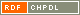# Show document

Title: Hamilton cycle and Hamilton path extendability of Cayley graphs on abelian groups Miklavič, Štefko (Author)Šparl, Primož (Author)http://dx.doi.org/10.1002/jgt.20621 English Not categorized 1.01 - Original Scientific Article IAM - Andrej Marušič Institute In this paper the concepts of Hamilton cycle (HC) and Hamilton path (HP) extendability are introduced. A connected graph ▫$\Gamma$▫ is ▫$n$▫-HC-extendable if it contains a path of length ▫$n$▫ and if every such path is contained in some Hamilton cycle of ▫$\Gamma$▫. Similarly, ▫$\Gamma$▫ is weakly ▫$n$▫-HP-extendable if it contains a path of length ▫$n$▫ and if every such path is contained in some Hamilton path of ▫$\Gamma$▫. Moreover, ▫$\Gamma$▫ is strongly ▫$n$▫-HP-extendable if it contains a path of length ▫$n$▫ and if for every such path $P$ there is a Hamilton path of ▫$\Gamma$▫ starting with ▫$P$▫. These concepts are then studied for the class of connected Cayley graphs on abelian groups. It is proved that every connected Cayley graph on an abelian group of order at least three is 2-HC-extendable and a complete classification of 3-HC-extendable connected Cayley graphs of abelian groups is obtained. Moreover, it is proved that every connected Cayley graph on an abelian group of order at least five is weakly 4-HP-extendable. graph theory, Hamilton cycle, Hamilton path, n-HC-extendable, strongly n-HP-extendable, weakly n-HP-extendable, Cayley graph, abelian group 2012 str. 384-403 Vol. 70, no. 4 0364-9024 519.17 10243597641296 79Document is not linked to any category.

Average score: (0 votes) Voting is allowed only to logged in users.Hover the mouse pointer over a document title to show the abstract or click on the title to get all document metadata.

## Secondary language

Language: English teorija grafov, Hamiltonov cikel, Hamiltonova pot, Cayleyjev graf, Abelova grupa